# Velocity-Time Graphs

GCSELevel 6-7Level 8-9AQACambridge iGCSEEdexcelOCRWJEC

## Velocity-Time Graphs

A velocity-time graph  (or speed-time graph) is a way of visually expressing a journey.

We are going to be using velocity-time graphs to find two things, primarily: total distance, and acceleration.

There are 5 key skills you need to learn

Make sure you are happy with the following topics before continuing:

Level 6-7GCSEAQAEdexcelOCRWJECCambridge iGCSE

## Velocity-Time Graphs – Key things to remember:

With speed on the $y$-axis and time on the $x$-axis, a speed-time graph tells us how someone/something’s speed has changed over a period of time.

1) The gradient of the line = Acceleration
3) Flat section means constant velocity (NOT STOPPED)
4) Area under the graph = Distance travelledLevel 6-7GCSEAQAEdexcelOCRWJECCambridge iGCSE

## Skill 1: Describing a graph

One Skill you will need learn is describing a velocity time graph.

Example: The speed-time graph shows a $50$-second car journey. Describe the $50$ second journey.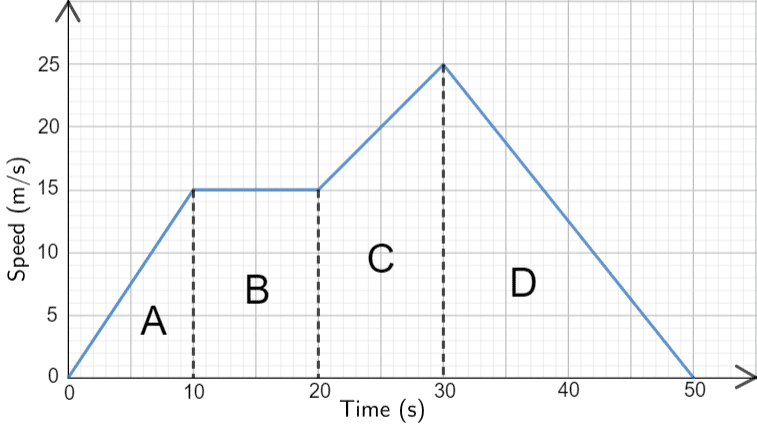Step 1: Split the graph up into distinct sections, these can be seen in the image as $A, B, C$ and $D$.

Step 2: In detail describe each part of the journey, ensuring to use numerical values throughout.

Section $A$ – The car accelerated from $0$ to $15$ m/s over the first $10$ seconds (because the line is straight, the acceleration is constant).

Section $B$ –  The line is flat, meaning the car’s speed did not change for $10$ seconds – meaning it was moving at a constant speed.

Section $C$ – The car accelerated up to $25$ m/s over the next $10$ seconds,

Section $D$ –  Finally it spent the last $20$ seconds decelerating back down to $0$ m/s.

Level 6-7GCSEAQAEdexcelOCRWJECCambridge iGCSE
Level 6-7GCSEAQAEdexcelOCRWJECCambridge iGCSE

## Skill 2: Calculating Acceleration

Acceleration is calculated as the change in speed over time.Example: The speed-time graph shows a $50$-second car journey, find which section of the graph has the greatest acceleration.

We know,

The gradient of the line = Acceleration

We must find the gradient of the each section.

Section $\bf{A}$:  Acceleration between $0$s and $10$s $=$ gradient$=\dfrac{15-0}{10-0}=1.5$ m/s$^2$

Section $\bf{B}$: This section is flat, meaning the acceleration will be $0$

Section $\bf{C}$: Acceleration between $20$s and $30$s $=$ gradient $=\dfrac{25-15}{30-20}=1$ m/s$^2$

Section $\bf{D}$: Acceleration between $30$s and $50$s $=$ gradient $=\dfrac{0-25}{50-30}=\dfrac{-25}{20} = -1.25$ m/s$^2$

Section $\bf{A}$ has the largest acceleration, so the maximum acceleration is $1.5$ m/s$^2$

Note: units of acceleration are expressed in distance/time$\bold{^2}$, which in this case is m/s$\bold{^2}$.

Level 6-7GCSEAQAEdexcelOCRWJECCambridge iGCSE

## Skill 3: Calculating total distance travelled

Calculating the total distance travelled is one of the most common exam questions you may see.Example: The speed-time graph shows a $50$-second car journey, Calculate the total distance travelled over the $50$ seconds.

we know,

Area under the graph = Distance travelled

To work out the area under this graph, we will break it into $4$ shapes: $A$, $B$, $C$, and $D$.

This gives two triangles, a rectangle, and a trapezium, which are all shapes that we can work out the area of.

$\text{A}=\dfrac{1}{2}\times10 \times15= 75$ m

$\text{B}=10\times15=150$ m

$C=\dfrac{1}{2}(15+25)\times10=200$ m

$D=\dfrac{1}{2}\times20\times25=250$ m

Total distance travelled:

$75+150+200+250=675$ m

Level 8-9GCSEAQAEdexcelOCRWJECCambridge iGCSE
Level 8-9GCSEAQAEdexcelOCRWJECCambridge iGCSE

## Skill 4: Average of curved graphs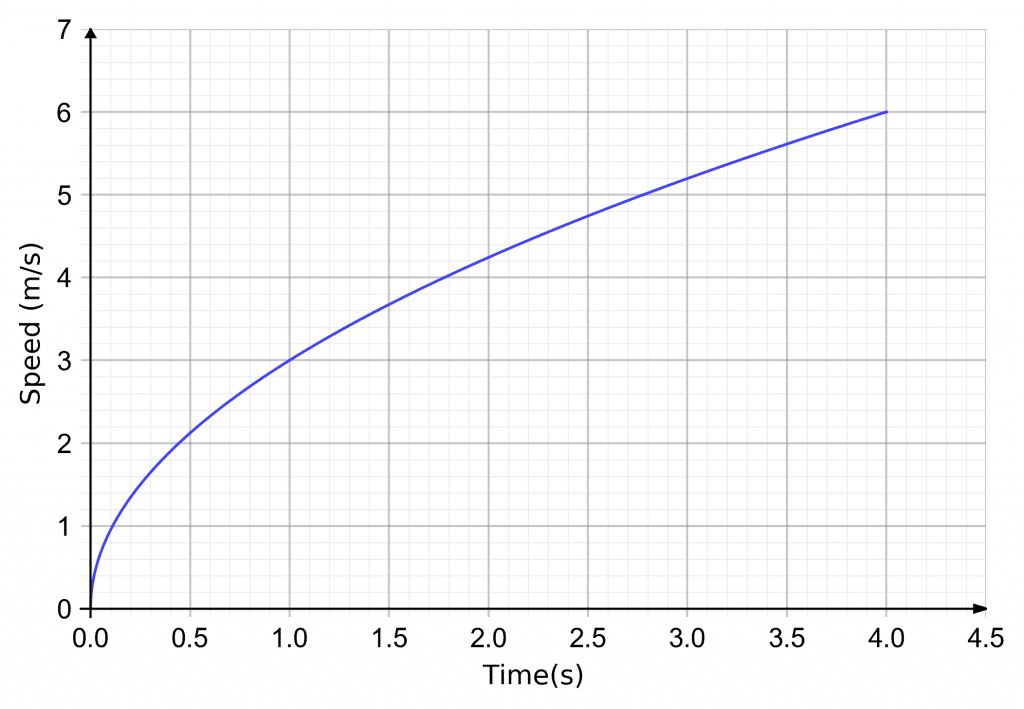Finding the average gradient, is the gradient over a length of time.

Example: A speed-time graph of the first $4$ seconds of someone running a race is shown.

Calculate the average acceleration over the $4$ seconds.

We know:

The gradient of the line = Acceleration

To work out the average acceleration over the $4$ seconds, we will draw a line from where the graph is at $0$ s to where the graph is at $4$ s and find the gradient of it.

So, we get the average acceleration to be,

$\text{gradient}=\dfrac{6-0}{4-0}=1.5$ m/s$^2$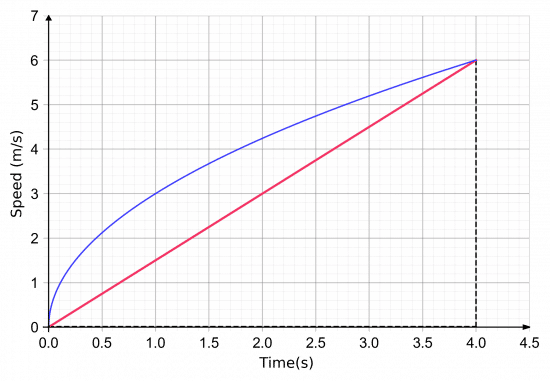Level 6-7GCSEAQAEdexcelOCRWJECCambridge iGCSE

## Skill 5: Instantaneous gradient of a curveFinding the instantaneous gradient, is the gradient of the tangent at a point.

Example: A speed-time graph of the first $4$ seconds of someone running a race is shown.

Calculate the instantaneous acceleration $2$ seconds in.

To do this we will draw a tangent to the line after $2$ seconds and work out the gradient of that. This is shown above.

Then, we get the instantaneous acceleration to be,

$\text{gradient}=\dfrac{5.8-3.2}{3.5-1.0} = 1.04$ m/s$^2$ ($3$ sf).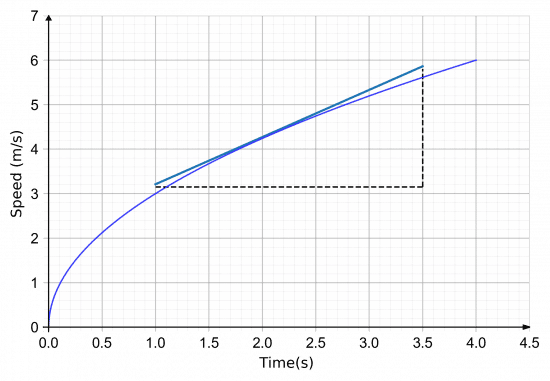Level 6-7GCSEAQAEdexcelOCRWJECCambridge iGCSE

## Velocity-Time Graphs Example Questions

So, we will firstly draw a straight from the origin to $(12, 4)$, since after $12$ seconds, it’s reached $4$ m/s. Then, for the next part we’re told the deceleration is $0.1$ m/s$^2$ for $20$ seconds. So, if the speed decreases by $0.1$ every second, after $20$ seconds it will be

$0.1 \times 20=2$ m/s

Therefore, by $32$ seconds in the speed is $2$ m/s, so we will draw a straight line from $(12, 4)$ to $(32, 2)$. Finally, a constant speed will be represented by a flat line that goes until the $50$ second point, still at $2$ m/s. The result should look like the graph below.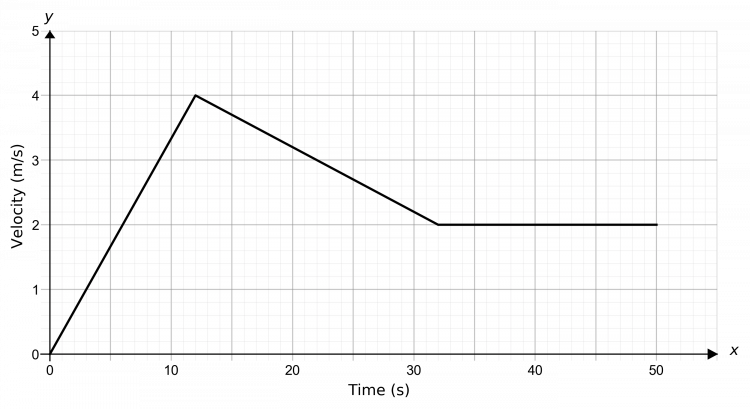Gold Standard Education

We need to find the area underneath the graph. To do this, we will split it up into shapes we know how to calculate the area of, as seen below.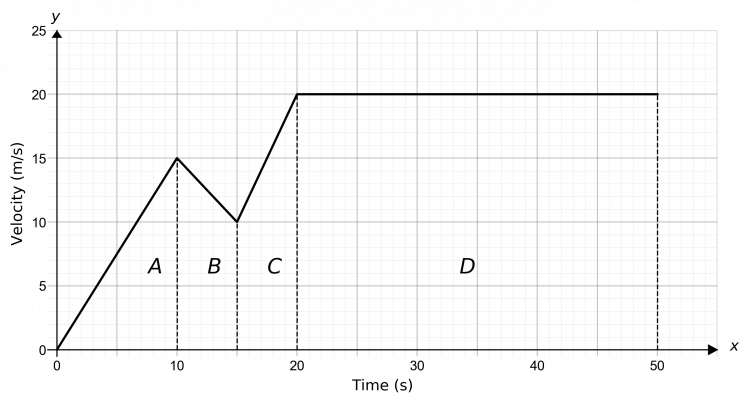$A$ is a triangle, $B$ and $C$ are trapeziums, and $D$ is a rectangle. So, we get

$\text{A}=\dfrac{1}{2}\times10\times15=75$ m

$\text{B}=\dfrac{1}{2}\times(10+15)\times5=62.5$ m

$\text{C}=\dfrac{1}{2}\times(10+20)\times5=75$ m

$\text{D}=30\times20 = 600$ m

Therefore, the total distance travelled by the cyclist is

$75+62.5+75+600=812.5$ mGold Standard Education

In order to determine the average acceleration, we draw a line from the origin to the endpoint of the graph, as seen below. The average acceleration is given by the gradient of this line.

Hence the average acceleration is,

$\text{gradient}=\dfrac{4-0}{50-0}= 0.08$ m/s$^2$Gold Standard Education

## Velocity-Time Graphs Worksheet and Example Questions

### (NEW) Velocity-Time Graphs Exam Style Questions - MME

Level 6-7Level 8-9GCSENewOfficial MMEGold Standard Education

Level 4-5GCSEKS3

Level 4-5GCSEKS3

Level 1-3GCSEKS3